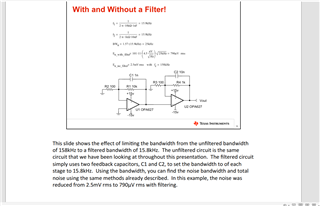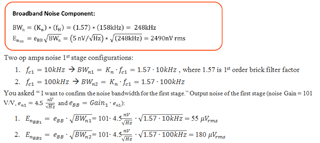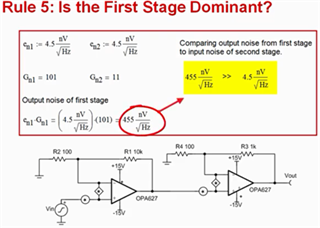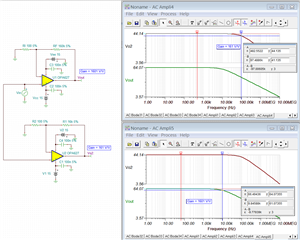This thread has been locked.

If you have a related question, please click the "Ask a related question" button in the top right corner. The newly created question will be automatically linked to this question.

# OPA627: Confirm the noise bandwidth for two stage

Part Number: OPA627

Hi Team,

I check the TI Precision Lab NOISE4. There are two stages Gain. I want to confirm the noise bandwidth for the first stage.For the above

(1), If f1=10K, f2=100K, In order to calculate En_with_filter that is the firs stage output noise in the second stage. BWn1=SQRT(1.57*10K), BWn2=SQRT(1.57*100K)

En_with_filter=101*11*4.5 nV/SQRT(HZ)* BWn1 Or En_with_filter=101*11*4.5 nV/SQRT(HZ)* BWn2.  Which is correct?

(1), If f1=100K, f2=10K, Then BWn1=SQRT(1.57*100K), BWn2=SQRT(1.57*10K),

En_with_filter=101*11*4.5 nV/SQRT(HZ)* BWn1 Or En_with_filter=101*11*4.5 nV/SQRT(HZ)* BWn2.  Which is correct?

• Hi Tengfei,

(1). If f1=10K, f2=100K, In order to calculate En_with_filter that is the first stage output noise in the second stage. BWn1=SQRT(1.57*10K), BWn2=SQRT(1.57*100K)

If I understand your inquiry correctly, you want " I want to confirm the noise bandwidth for the first stage.". Below is the calculation.

(Note: Assumed Gain = 101 V/V. If you are using the same OPA627 op amp in the example, Gain will be different in f1=10kHz and f2=100kHz due to GBP).Regarding to your second questions, you may calculate with the same method. However, you need to taken into account of Noise Gains with different cutoff frequency configurations.

In the Op Amp Noise video presentation in 8.4 or  TI Precision Lab NOISE4. Rule #5 demonstrates that you want to establish the dominant noise in the 1st op amp stage. If you follow the rule, you may safely ignore the noise gain on the 2nd stage as shown in the image below (simplify the noise gain calculation).If your questions are: 1st op amp stage with fc1 =10kHz and 2nd op amp stage with fc2 = 100kHz:

If the first stage fc1 = 10kHz, then the 1st gain stage in OPA627 is approx. 16MHz/10kHz = 1600 V/V (use GBP rule assume a single pole roll off)

If the second stage fc2 = 100kHz, the 2nd gain stage in OPA627 is approx. 160V/V.

In your second inquiry:

If the 1st stage fc1 = 100kHz, the 1st gain stage in OPA627 is approx. 160V/V.

If 2nd stage fc2 = 10kHz, the 2nd gain stage in OPA627 is approx. 1600 V/V.

We recommended to implement Rule #5 in the noise gain presentation. If you do that, you may only require one precision low noise op amp (instead of 2) which it may lower the overall cost and simplify the noise calculation or estimation.

If you have additional questions, please let us know.

Best,

Raymond

• Hi Ray,

My point is that there are still 2 OPA627 like I post in the picture. I want to confirm the En_with_filter that is the firs stage output noise in the second stage

and how to confirm the first stage noise bandwidth in the second stage. Not compare the different fc, the different output noise.

Because if there is 2-level in-phase amplification, the circuit has 2 fc. I don't know if the 2 fc is not equal, how to calculate the irst stage noise bandwidth in the second stage?

Could you help me to confirm my question?

• Hi Tengfei,

If you provide me with the cutoff frequencies in the op amp stage, I would calculate the total noise for you.

Since you only provided the cutoff frequency, you will have two different gains for the first and second stages with and without Cf capacitor filter.

For instance, fc1=10kHz, its OPA627's gain is 1601 V/V or 64.07dB (10^(64.07/20); fc2 = 100kHz, its OPA627's gain is 161V/V or 44.14dB (10^(44.14/20))OPA627 Noise Simulation 10182021.TSC

Both total noise calculations are incorrect, because op amp gains are not 101 V/V and 11V/V per the broadband frequency. If you rephrase your questions, I will calculate the total noise of the circuit.

It will be better to provide me with feedback gain resistors and capacitors of your circuits rather than the cutoff frequency as posted in f1=100K, f2=10K.

Best,

Raymond